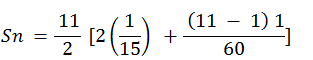Guru

# Find the sum of the following APs.(iv) 1/15, 1/12, 1/10, …… , to 11 terms Q.1(4)

• 0

How to solve the problem of the arithmetic progressions of exercise 5.3 of math of class 10th . This is very important question how i solve this problem Find the sum of the following APs.(iv) 1/15, 1/12, 1/10, …… , to 11 terms

Share

1. Given, 1/15, 1/12, 1/10, …… , to 11 terms

For this A.P.,

First term, a = 1/5

Common difference, d = a2 –a1 = (1/12)-(1/5) = 1/60

And number of terms n = 11

We know that, the formula for sum of nth term in AP series is,

Sn = n/2 [2a + (n – 1) d]= 11/2(2/15 + 10/60)

= 11/2 (9/30)

= 33/20

• 0